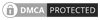## The long way - by hand

In this article, will demonstrate some of the basics for carrying out fire sprinkler calculations by the long hand method with just the aid of a scientific calculator or our own hydraulic calculator - Hcal2 which you can freely download from our website.

We will for this example use simple three sprinklers and three pipes which would of course be part of a much larger fire sprinkler system. These basic procedures can also be used for calculating many other types of systems such as fire hydrant, hose reel or the discharge from a water cannon or monitor we can also use the same principal for almost all other water-based fire protection systems if we have a k-factor for the output device (fire sprinkler, water mist nozzle and so on).

In this example, will we use a very simple system with just three sprinklers and three pipes this is often called a range pipe or branch pipe, which is part of a larger 'tree system'. A tree system is 'end feed', that is water is only fed from one direction as opposed to a grid or loop system when water may arrive at the sprinkler head from more than one direction.

Below is a diagram of the three sprinklers and three pipes which we will calculate.  We have dimensioned the pipe lengths and given each junction point a unique node reference number which we use throughout the calculations.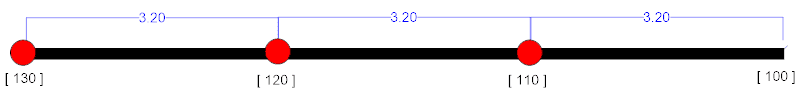For each pipe, we need to know the pipe length, internal diameter (ID) of the pipe and the pipe material so we can determine the pipes c-factor, the table below summarises the pipe data which we will need for the calculation for this example:

 Node Ref Pipe Size ID (mm) Length (m) C-factor 130-120 27.30 3.20 120 120-110 27.30 3.20 120 110-100 36.00 3.20 120

We will also we will need some additional information such as the type sprinkler head, the area each head is covering, the design density for each sprinkler head in the system.

For this example, we will use the following design parameters:

design density: 7.50 mm/min
sprinkler head: K-factor of 70 with a minimum pressure 0.5 bar

In this example, we have kept it very simple and used the same sprinkler head for all three sprinklers but this may not always be the case so again it may be useful to summarises the information in a table such as this:

 Node Ref Design Density(mm/min) Sprinkler k-factor Sprinkler minimumpressure (Bar) Head area (m2) 130 7.50 70 0.5 10.20 120 7.50 70 0.5 10.20 110 7.5 70 0.5 10.20

The first step is to calculate the minimum flow which will be required at the most remote sprinkler which in this case is at node , this is a two-step process as will need to calculate the minimum flow required to satisfy the 7.50 mm/min design density and then find the flow rate from the sprinkler given the sprinklers minimum pressure requirement, whichever is the greater flow will become our initial flow from the first sprinkler at node .

We will first calculate the flow given the design density of 7.50 mm/min and the area the head is covering, we do this by multiplying the design density by the head area:

Equation 1:

q1 = (design density) x (area per sprinkler)

In this example, this gives:

q1 = 7.50 mm/min x 10.20 m2 = 76.50 L/min

The second step is to calculate the minimum flow from the sprinkler given the K-Factor and the minimum head pressure by using the standard K-Factor formula:

Equation 2:

q = kp0.5

Where

p = the required pressure
q = the required flow from the first sprinkler
k = the discharge coefficient of the sprinkler (k-factor)

In this example, this gives:

q = 70 x 0.50.5 = 49.50 L/min

By comparing the two calculations above we can see that the minimum flow required from the sprinkler head will be 76.50 L/min as this is the highest flow rate from the two calculations and is required to meet the 7.50 mm/min design density.  We can also see that the minimum sprinkler pressure of 0.5 bar is not sufficient to produce the required flow rate so the next step will be to determine what pressure will be required to produce the required flow of 76.50 L/min at the first sprinkler head at node  we can do this by using equation 3.

Equation 3

p = (q/k)2

In the example, this gives:

p = (76.50 / 70)0.5 = 1.194 bar

We have now determined the minimum pressure and flow for the first sprinkler at node  which will be 76.50 L/min @ 1.19 bar the next step is to calculate the pressure drop in the pipe between node  and  and for this we will use the Hazen-Williams pressure loss formula.

Equation 4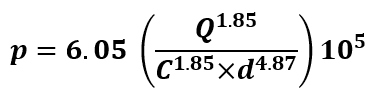Where

p = pressure loss in bar per meter
Q = flow through the pipe in L/min
C = friction loss coefficient
d = internal diameter of the pipe in mm

We know that the flow rate from the sprinkler at node  is 76.50 L/min and this will be the flow rate in the first pipe between nodes -. As the pipe has an internal diameter of 27.30 mm and has a C value of 120 this will give us: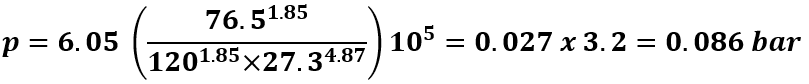The pressure loss in the first pipe is 0.027 Bar/m and the total pressure loss in the pipe is 0.086 bar.

We now need to add the pressure loss in the pipe to the start pressure at the sprinkler head at node  which was 1.19 bar to find to pressure at node  and at the seconded sprinkler head at node  this gives us 1.194 + 0.086 = 1.28 bar.

The next step is to find the flow from the seconded sprinkler head at node  to do this we will use the K-Factor formula

Equation 5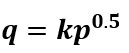This gives 70 x 1.2800.5 = 79.20 L/min from the sprinkler head at node  which we now add to the flow in the first pipe node - to find the total flow in the second pipe - to find the total flow in the seconded pipe which is 155.70 L/min.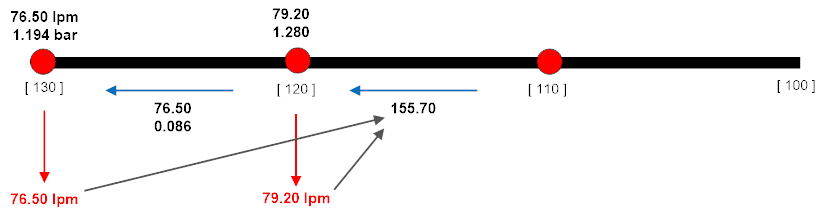Having found the total flow in the seconded pipe - we can now find the pressure loss in, to do this we will use the Hazen-Williams pressure loss, formula 4 which we used above this gives us: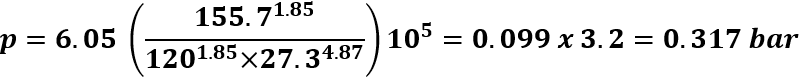We now add the pressure loss 0.317 bar to the pressure at node  to find the pressure at node  this give us: 0.317 + 1.280 = 1.597 bar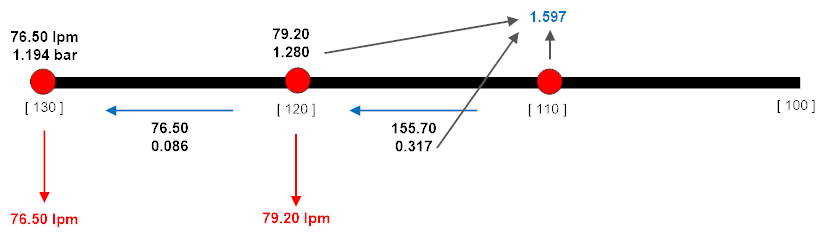We now need to find the flow from the sprinkler at node  we do this by using the k-factor given in equation 5 as we now know the pressure at node  is 1.597 bar, this gives 70 x 1.5970.5 = 88.50 L/min from the sprinkler head at node .  We now add this flow to the flow in the seconded pipe - to find the total flow in the third pipe - which will give us the flow of 244.20 L/min.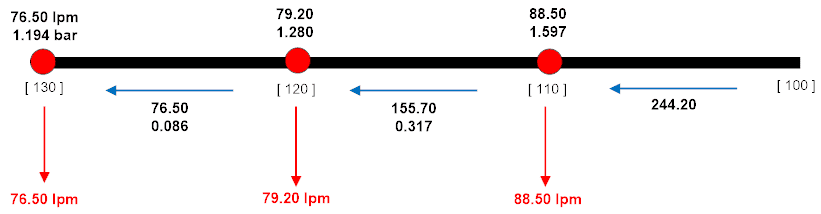The last step is to find the pressure loss in the third pipe - and again we will use the Hazen-Williams pressure loss formula given is formula 4 above. However, the last pipe has an internal diameter of 36.0 mm so this gives us: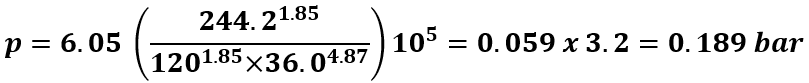We now add the pressure loss in this pipe to the pressure at node  to find the pressure at node  this will be 0.189 + 1.597 = 1.786 bar.  We have now completed the calculation for all three sprinkler heads and have found the source pressure and flow required for this system is:

244.20 L/min @ 1.786 Bar

This pressure and flow is often referred to as the source requirement for the system and is the minimum pressure and flow required for the system for it to be able to provide the required design density (in this example 7.50 mm/min) at the most remote head [MRH] at node .

You should also be able to see that only the Most Remote Head has the minimum requirement of 7.50 mm/min design density and all the other sprinklers will have a higher pressure as they are hydraulically closer to the water source so they will have a higher pressure and will discharge more water through the sprinkler this can be seen in the table below:

 Node Ref min Design Density(mm/min) Pressure (Bar) Flow from sprinkler(L/min) Head Area(m2) ActualDesign Density 130 [MRH] 7.50 1.194 76.50 10.20 7.50 120 7.50 1.280 79.20 10.20 7.76 130 7.50 1.597 88.50 10.20 8.68

Sprinkler calculation step by step

1. Calculate minimum flow from the MRH with the sprinkler minimum pressure and k-factor
2. Calculate the minimum flow given the system design density and sprinkler head area.
3. If the calculation in step 2 is the highest flow demand, then calculate the required head pressure otherwise we can use the minimum sprinkler pressure in step 1.
4. Calculate the pressure loss in the pipe.
5. Add the head pressure to the pressure loss in step 4 to determine the pressure at the next sprinkler.
6. Use the k-factor formula to determine the flow from the sprinkler head.
7. Repeat step 4 to 6 until you do not have any more sprinklers or pipesCanute LLP
15 Queen Square
Leeds, West Yorkshire
United Kingdom LS2 8AJ

t: +44 (0) 113 328 0350
e: info@canutesoft.com

 Copyright © 2021, Canute LLPRegistered in England & Wales, Partnership No. OC305985